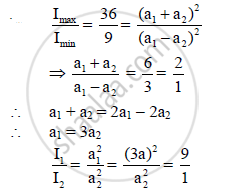Share

# In Young’S Experiment, the Ratio of Intensity at the Maxima and Minima in an Interference Pattern is 36 : 9. What Will Be the Ratio of the Intensities of Two Interfering Waves? - Physics

#### Question

In Young’s experiment, the ratio of intensity at the maxima and minima in an interference
pattern is 36 : 9. What will be the ratio of the intensities of two interfering waves?

#### SolutionThe ratio of the intensities of the two interfering waves is 9 : 1.

Is there an error in this question or solution?